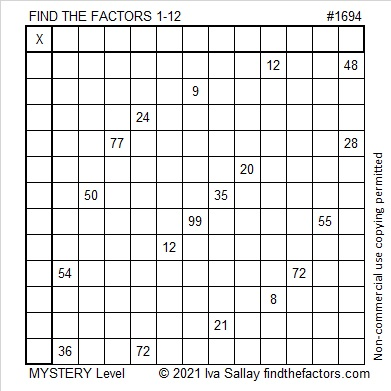# 1694 Football Game Day

### Today’s Puzzle:

Today all over the United States family and friends will gather to watch or play a game of football. If you would like to change things up a little, here’s a game ball for you to practice multiplication and division facts. Just write the numbers from 1 to 12 in both the first column and the top row so that those numbers and the given clues form a multiplication table. Some clues might be tricky, but enough of them aren’t that I am confident you can score with this football!Here’s the same puzzle without any distracting color:### Factors of 1694:

• 1694 is a composite number.
• Prime factorization: 1694 = 2 × 7 × 11 × 11, which can be written 1694 = 2 × 7 × 11².
• 1694 has at least one exponent greater than 1 in its prime factorization so √1694 can be simplified. Taking the factor pair from the factor pair table below with the largest square number factor, we get √1694 = (√121)(√14) = 11√14.
• The exponents in the prime factorization are 1, 1, and 2. Adding one to each exponent and multiplying we get (1 + 1)(1 + 1)(2 + 1) = 2 × 2 × 3 = 12. Therefore 1694 has exactly 12 factors.
• The factors of 1694 are outlined with their factor pair partners in the graphic below.### More About the Number 1694:

From OEIS.org we learn that 1694³ = 4,861,163,384, a number that uses each of the digits 1, 3, 4, 5, and 8 exactly twice.